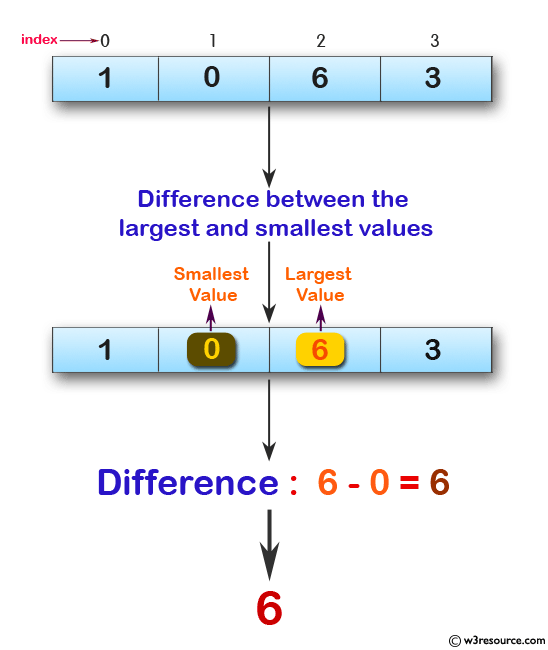﻿ Swift Array Programming Exercise: Find the difference between the largest and smallest values in a given array of integers and length 1 or more - w3resource# Swift Array Programming Exercises: Find the difference between the largest and smallest values in a given array of integers and length 1 or more

## Swift Array Programming: Exercise-25 with Solution

Write a Swift program to find the difference between the largest and smallest values in a given array of integers and length 1 or more.

Pictorial Presentation:Sample Solution:

Swift Code:

``````func value_diff(nums: [Int]) -> Int {
var min_num = nums
var max_num = nums

for x in 0..<nums.count
{
min_num = min(nums[x], min_num)
max_num = max(nums[x], max_num)
}

return max_num - min_num
}

print(value_diff(nums: [-5, -3, -7, 0]))
print(value_diff(nums: [8, 2, 14, 24]))
print(value_diff(nums: [1, 0, 6, 3]))
```
```

Sample Output:

```7
22
6
```

Swift Programming Code Editor:

Improve this sample solution and post your code through Disqus

What is the difficulty level of this exercise?

﻿